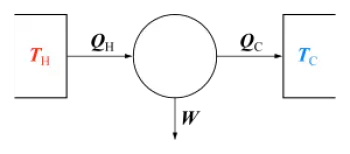# Third Law of ThermodynamicsThe third law of thermodynamics, also called Nernst's theorem, is a theorem of thermodynamics.

This principle states that the entropy of a system at the absolute zero temperature is a well-defined constant. This is because, at the absolute zero temperature, a system is in a basic state, and entropy increases are achieved by degeneration from this basic state.

## Formulations of the Third Principle of Thermodynamics

Like the second principle of thermodynamics, to which it is closely linked, this establishes the impossibility of realizing a certain class of phenomena. There are several formulations, a modern one is the following:

"The entropy of a perfect crystal at absolute zero is exactly equal to 0."

At absolute zero (zero kelvin), the system must be in a state with the least possible energy, and the statement about the third law of thermodynamics states that a perfect crystal at this minimum temperature only has a possible microstate. Entropy is in fact from a statistical point of view proportional to the logarithm of the number of accessible microstates, and for a system consisting of many particles, quantum mechanics indicates that there is only a single state called the ground state with the minimum energy . If the system does not have a precise order (for example, if it is an amorphous solid)), then, in practice, a finite entropy remains even at absolute zero, but the low temperature system remains blocked in one of the many configurations that have minimal energy. The residual constant value is called the residual entropy of the system.

The formulation of this principle according to Nernst-Simon refers to thermodynamic processes at constant low temperature:

"The entropy change associated with each reversible isothermal phase transformation is reduced to zero, since the temperature at which the process takes place goes to 0 K."

In this case, a condensed liquid or a solid means a condensed system.

Nernst's classic formulation (currently considered more a consequence of the third law of thermodynamics instead of the law itself) states:

"It is not possible for any process, even idealized, to reduce the entropy of a system to its absolute zero value through a finite number of operations (ie, thermodynamic transformations). It was demonstrated in 2017 by Masanes and Oppenheim »

There is also a formulation of the third law that deals with the specific behavior of energy:

"If the set of two thermodynamic systems is isolated, then an exchange of energy between them has a finite value"

Author:

Published: May 23, 2018
Last review: May 22, 2018